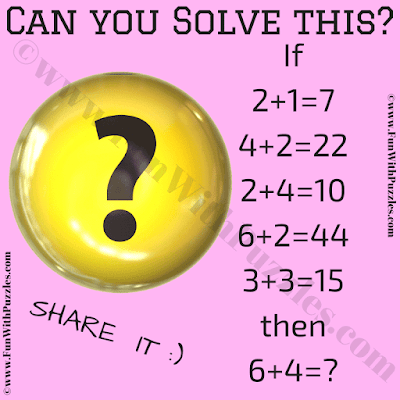This is a very interesting mathematical reasoning puzzle in which your challenge is to crack the code. In this code-cracking puzzle, you are given some number equations. Your challenge in this puzzle is to crack the logical or mathematical pattern in these number equations. Once you are able to crack the code, find the value of the missing number which will replace the question mark. So, can you crack the code?Can you crack the code to find the missing number?

The answer to this "Mathematical Reasoning Crack the Code Puzzle", can be viewed by clicking on the answer button.